## LetsPlayMaths.Com

WELCOME TO THE WORLD OF MATHEMATICS

# Class 3 Subtraction

Class II Recap

Subtraction Without Borrowing

Subtraction with Borrowing

Story Time Subtraction

Addition and subtraction

Subtraction Test

Subtraction Worksheet

Answer Sheet

## Class II Recap

In Standard two, we learnt how to subtract 3-digits numbers with or without borrowing.
Few examples are shown below.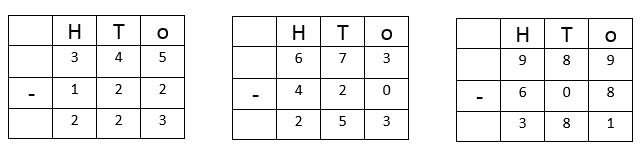## Subtraction Without Borrowing

The same 3-digit numbers subtraction method is applied to 4-digits, 5-digits and 6-digits.
Let us learn 4-digits or 5-digits subtraction without borrowing. Few examples are given below.

Example 1. Subtract 6027 from 7649

Solution. Putting numbers in column format as shown in below figure.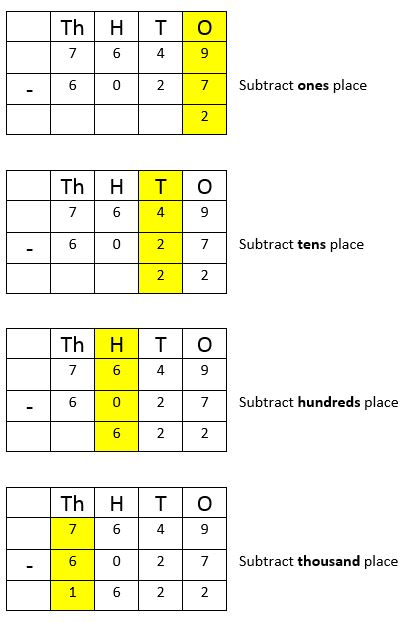7649 – 6027 = 1622
So, the answer is 1622

Example 2. Subtract 21731 from 54896.

Solution. Putting numbers in column format as shown below.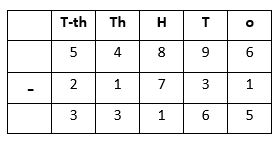54896 - 21731 = 33165
So, the answer is 33165.

## Subtraction with Borrowing

We have already learnt the subtraction with borrowing on 2 and 3 digit numbers. We will use the
same method for addition 4 and 5 digit numbers. Few examples are given below.

Example 1. Subtract 29627 from 76322.
Solution. Put the numbers in column format and subtract.
Step 1.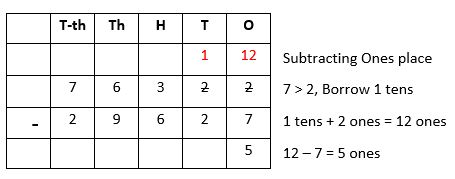Step 2.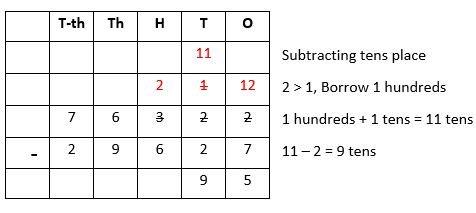Step 3.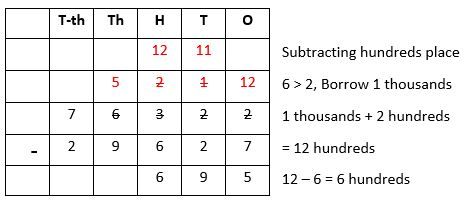Step 4.Step 5.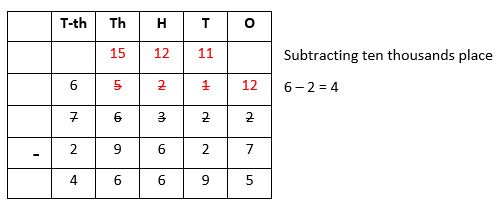76322 – 29627 = 46695
So, the answer is 46695.

## Story Time Subtraction

In our day to day life we use concept of subtraction in many ways, few examples are shown below.

Example 1. There are 6500 persons in a stadium, due to rain 2354 persons left the stadium.
How many persons are present in the stadium to see the match?
Solution.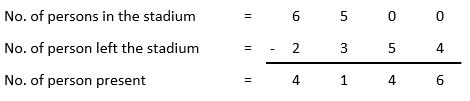There are 4146 persons present in the stadium.

Example 2. Total population of a town is 75575, out of which 37895 are men and remaining are women. How many women are leaving in the town?

Solution.So, there are 37680 women leaving in the town.

## Addition and subtraction

In our day to day life we use concept of both addition and subtraction in many ways.
Few examples are given below.

Example 1. In a garden there are 25000 trees, out of these 12615 are apple trees, 1567 are orange trees and the remaining are mango trees. How many are mango trees?

Solution.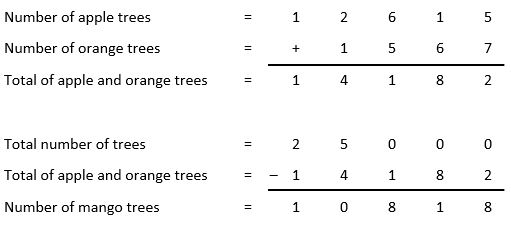## Class-3 Subtraction Worksheet

Subtraction Test - 1

Subtraction Test - 2

## Class-3 Subtraction Worksheet

Subtraction Worksheet - 1

Subtraction Worksheet - 2

Subtraction Worksheet - 3

Subtraction Worksheet - 4

## Answer Sheet

Subtraction-AnswerDownload the pdf

Copyright © 2021 LetsPlayMaths.com. All Rights Reserved.